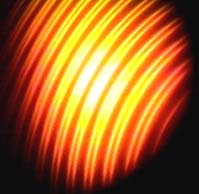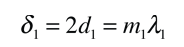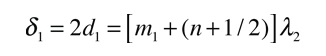# Sodium Doublet on Fabry-Perot

The image at left below shows the appearance of the interference fringes of the sodium doublet produced by Fabry-Perot optics. This is the appearance at one position. As the micrometer is adjusted to increase the mirror spacing, the appearance changes as the fringes merge together. If the micrometer is advanced until the fringes look the same again, then you have advanced one of the fringe sets one full order with respect to the other. This allows you to calculate the wavelength difference. The video at right shows the progression which is used to determine the wavelength difference.Fabry-Perot geometry Fabry-Perot resolution
Index

Interference concepts

Example of sodium doublet

 HyperPhysics***** Light and Vision R Nave
Go Back

# Doublet Separation, Fabry-Perot

The resolvance of the Fabry-Perot interferometer is sufficient to resolve closely spaced spectral doublets like the sodium doublet and the hydrogen-deuterium doublet.

The experimental procedure yields a distance of movement of the interferometer mirror which allows the doublet separation to be calculated from the relationshipShow
The average wavelength of the doublet must be known from other measurements to make use of this relationship.
 For average wavelength = λ == nm
 and interferometer carriage movement = mm
 the doublet separation is == nm
 Fabry-Perot resolution
Index

Interference concepts

 HyperPhysics***** Light and Vision R Nave
Go Back

# Doublet Separation Expression

Subraction of the two distances obtained in the doublet experiment gives the following expression:This expresses the fact that the shorter wavelength has gained an order on the longer wavelength. Combining terms givesSubstituting this back into the top equation givesThe relationship for the doublet separation is thenCalculation
 Fabry-Perot interferometer Calculation
Index

Interference concepts

 HyperPhysics***** Light and Vision R Nave
Go Back

# Doublet Separation, Experiment

The experimental procedure involves examining the interference fringes of the light at normal incidence ( angle = 0°) such that constructive interference is produced by one wavelength of the doubletwhile the other wavelength is adjusted to a half-integer condition. This can be seen in the interference pattern as a weaker ring half-way between the stronger interference maxima. This corresponds toAdvancing the micrometer until this condition occurs again provides data from which the doublet separation can be calculated.Show doubletexpression
 Fabry-Perot interferometer
Index

Interference concepts

 HyperPhysics***** Light and Vision R Nave
Go Back